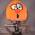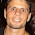## Sunday, 25 November 2012

### More accurate thermistor tables

A couple of weeks ago I wanted to create some thermistor tables for Marlin. At that time it had a copy of createTemperatureLookup.py, which I think was written by Zach Smith based on my article "Measuring temperature the easy Way". It uses the simple two constant thermistor equation based on the resistance at 25°C and beta.

The two constant formula was adequate for my own software because I have separate constants for each thermistor that I use and I calibrate them against a thermocouple at the working temperature and room temperature. Marlin however has a thermistor table for each type of thermistor, so if you use the same thermistor for the bed and the hot end they share a table. The problem with the simple equation is that beta is not very constant and depends on temperature. There are several values given on the datasheet for different temperature ranges
, none of them very applicable to our application. If you have beta correct for the hot end at say 250°C it is about 7°C out at the bed temperature, say 130°C.

I decided to make a new script which uses the three constant Steinhart–Hart equation. The graph shows the difference between the two equations over a large temperature range: -
The two constant equation is only accurate around the two temperatures the constants are calculated at (in this case 25°C and 256°C). When these are at opposite ends of the thermistors range the error in the middle is quite large.

The script I made is MakeTempTable.py. Its parameters are resistances at three temperatures. The tables is makes look like this: -

    {     344,       300     }, // r=   101 adc=  21.47
{     369,       295     }, // r=   108 adc=  23.08
{     397,       290     }, // r=   117 adc=  24.83
{     428,       285     }, // r=   126 adc=  26.75
{     461,       280     }, // r=   136 adc=  28.84
{     498,       275     }, // r=   147 adc=  31.12
{     538,       270     }, // r=   160 adc=  33.63
{     582,       265     }, // r=   173 adc=  36.37
{     630,       260     }, // r=   188 adc=  39.38
{     683,       255     }, // r=   205 adc=  42.69
{     741,       250     }, // r=   223 adc=  46.32
{     805,       245     }, // r=   243 adc=  50.31
{     875,       240     }, // r=   266 adc=  54.71
{     953,       235     }, // r=   290 adc=  59.55
{    1038,       230     }, // r=   318 adc=  64.88
{    1132,       225     }, // r=   349 adc=  70.77
{    1236,       220     }, // r=   384 adc=  77.26
{    1351,       215     }, // r=   423 adc=  84.42
{    1477,       210     }, // r=   466 adc=  92.32
{    1617,       205     }, // r=   515 adc= 101.05
{    1771,       200     }, // r=   570 adc= 110.68
{    1941,       195     }, // r=   632 adc= 121.30
{    2128,       190     }, // r=   702 adc= 133.01
{    2335,       185     }, // r=   782 adc= 145.91
{    2562,       180     }, // r=   872 adc= 160.11
{    2811,       175     }, // r=   975 adc= 175.70
{    3085,       170     }, // r=  1092 adc= 192.81
{    3384,       165     }, // r=  1225 adc= 211.53
{    3711,       160     }, // r=  1378 adc= 231.95
{    4066,       155     }, // r=  1554 adc= 254.15
{    4451,       150     }, // r=  1756 adc= 278.21
{    4866,       145     }, // r=  1989 adc= 304.15
{    5312,       140     }, // r=  2258 adc= 331.99
{    5787,       135     }, // r=  2570 adc= 361.68
{    6290,       130     }, // r=  2934 adc= 393.15
{    6820,       125     }, // r=  3357 adc= 426.25
{    7373,       120     }, // r=  3852 adc= 460.80
{    7945,       115     }, // r=  4433 adc= 496.54
{    8531,       110     }, // r=  5116 adc= 533.16
{    9125,       105     }, // r=  5921 adc= 570.31
{    9722,       100     }, // r=  6875 adc= 607.60
{   10314,        95     }, // r=  8007 adc= 644.61
{   10895,        90     }, // r=  9356 adc= 680.92
{   11458,        85     }, // r= 10968 adc= 716.13
{   11998,        80     }, // r= 12903 adc= 749.86
{   12509,        75     }, // r= 15234 adc= 781.80
{   12987,        70     }, // r= 18051 adc= 811.66
{   13428,        65     }, // r= 21469 adc= 839.27
{   13832,        60     }, // r= 25635 adc= 864.50
{   14197,        55     }, // r= 30732 adc= 887.30
{   14523,        50     }, // r= 36995 adc= 907.68
{   14811,        45     }, // r= 44725 adc= 925.72
{   15064,        40     }, // r= 54309 adc= 941.52
{   15284,        35     }, // r= 66249 adc= 955.23
{   15472,        30     }, // r= 81195 adc= 967.02
{   15633,        25     }, // r=100000 adc= 977.08
{   15769,        20     }, // r=123783 adc= 985.58
{   15883,        15     }, // r=154025 adc= 992.71
{   15978,        10     }, // r=192694 adc= 998.64
{   16057,         5     }, // r=242427 adc=1003.54
{   16121,         0     }, // r=306773 adc=1007.56


The ADC values in the table are multiplied by 16 because Marlin uses oversampling to give four more bits of precision. The old tables just multiplied the integer ADC value by 16 but I multiply it before rounding it to an integer so the table has the same precision as the oversampled ADC reading.

The script can also take ADC values as parameters instead of resistances. This allows you to calibrate a thermistor in situ. If you set the temperature to a value in an existing table and let it settle and then measure it with a thermocouple you know that the ADC value for the measured temperature is the value in the table for the set temperature. You can then produce a new more accurate table.

Two days after I put it on Github ErikZalm added a new script to the official version of Marlin to do exactly the same thing: createTemperatureLookupMarlin.py, amazing coincidence! It is different code but I think it uses exactly the same maths to find the three coefficients using simultaneous equations that I lifted from here

1.Hello Nophead, nice post.

Next time I will check your github before I rewrite a tool.
There where some users on IRC that had problems with the thermistor accuracy. That is why I rewrote this tool.
Your version is much cleaner. I replace mine.

Erik van der Zalm

2.When I work with thermistors at work, I usually use the B parameter equation. http://en.wikipedia.org/wiki/Thermistor#B_or_.CE.B2_parameter_equation

I would think with that equation you could pass the datasheet parameters, Beta, Resistance at a temperature, and the temperature that was measured at. In your table I'm not sure what Beta is, but the other two parameters would be 100k and 25. That's a good way to get an initial table, but your method may be better in the end for getting something that's well calibrated.

3.Apparently hadn't set up my profile, the "Unknown" author above is me.

4.Thav,
The whole point of the article is that the B parameter equation is not accurate enough over a wide range and the data sheet values are only given for 0-100, 20-100 and 20-80. To work at 240C you have to work out your own beta and then it isn't accurate at half that temperature. Steinhart–Hart is needed for a accuracy over a wide range.

5.When I was building the temperature sensor for my aquarium I got tired of playing with temperature tables. I was looking for something with decimal precision and it is a nightmare to achieve that with cheap NTC thermistors and temperature tables. Then I ended up moving to 1Wire temperature sensors, which don't require a table and are very precise.
Do you think it is worth the try?

6.The 1wire protocol is difficult to implement in firmware without screwing up the stepper motor timing or vice versa. Techzone used one and it caused lots of problems.

The main limit on precision for Reprap is the 10 bit ADC.

7.Great post as always! However there is one problem with the table. To generate it, the inverse equation is used i.e. in fact the script calculates the resistance based on the temperature. Unfortunately, the inverse equation can't be solved for all the inputs, because (B/3C)**3 + y**2 can turn negative and sqrt will be undefined in real numbers. May be that doesn't happen for Epcos thermistors, but happened to mine. So I guess one should put some effort into measuring the two resistances as precise as possible to the inverse equation work. Another option is to iterate through some range of resistances (the range could be "guessed") and calculate corresponding temperatures.

1.Are you sure you didn't make a mistake with your figures? I think it should work for all real thermistors.

8.Yeah, I am quite sure. For example, if I run the script with all the same input values only changing one temperature value by one degree or even a bit less I get sqrt undefined error. I think it is easy to be one degree off with the measuring equipment most of us have unless you are willing to conduct much more tests averaging the results. In fact, I made lots of failed attempts before I got it to work in the end. Of course, my thermistor is making things worse. It is an old model with quite a range for Beta (4500-7000) so it is harder to be on spot. But my whole point was more about the possible issue with using inverse equation, rather than difficulties of measuring and using the script.

1.Maybe it's a bug. If the inverse equation fails the implication is that no matter what the resistance is it never results in a particular temperature, which doesn't make sense for a real thermistor.

I can only assume the first step goes wrong, which is solving three simultaneous equations to find the coefficients.

Please can you give some example values that work and some that don't for the same thermistor. The interesting thing would be to see the graph of T against R in the case that fails.

2.I guess it is impossible to insert pictures into replies so here is the link: http://andrey2345.blogspot.ru/

3.I suspect that it is because you haven't used 25C for T0/R0. It seems that the low temperature part of the curve is very inaccurate and perhaps R becomes infinite before T gets to 0.

4.Hi! I got the same problem, and I found the problem to be an extra equal sign on line 76:
r2 == resistance(int(arg))
It should be:
r2 = resistance(int(arg))

Actually I had one other issue :
When using --adc parameters, --rp must be set first to have effect. The default is 4.7k which is correct for most boards, but I have a melzi with a 10k pullup...

Now I have a very accurate table, thanks Nop head!

9.I haven't because the datasheet's T0 is 150C ;) But I don't need the lower part of the curve anyway.

If you run the script you'll see that it fails with an error immediately. Remember it iterates from 300 and down. So it never gets to the lower part of the temperature range.

10.Is this sample table for the Epcos B57861S104F40 100K 1% thermistor from the Mendel90? https://github.com/nophead/Mendel90/blob/master/dibond/bom/bom.txt

1.No that is the bed thermistor and it doesn't go up to 300C. I think the table above is for the extruder thermistor. You can find them both in my fork of Marlin on Github.